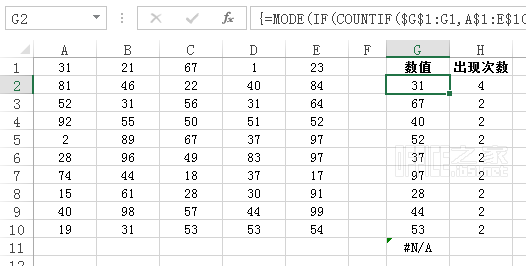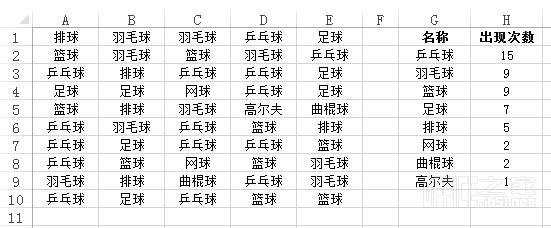Excel将区域中重复数据按出现次数的多少降序排序提取到一列=MODE(IF(COUNTIF(\$G\$1:G1,A\$1:E\$10)=0,A\$1:E\$10))

MODE函数

Number1：是要计算众数的第一个数字引数。

Number2：可选参数，是第2 个到第255 个您要计算众数的数字引数。也可以使用单一阵列或阵列参照，来取代以逗点分隔的引数。Sub 按出现次数排序()
Dim d As Object
Dim Arr
Dim i As Integer, j As Integer
Application.ScreenUpdating = False

'提取不重复值并计算出现次数
Set d = CreateObject("Scripting.Dictionary")
Arr = Range("A1:E10")
For i = 1 To UBound(Arr, 1)
For j = 1 To UBound(Arr, 2)
If Not d.Exists(Arr(i, j)) Then
Else
d.Item(Arr(i, j)) = d.Item(Arr(i, j)) + 1
End If
Next
Next

'输出并排序
Range("G2").Resize(d.Count) = Application.Transpose(d.keys)
Range("H2").Resize(d.Count) = Application.Transpose(d.items)
Range("G2:H2").Resize(d.Count).Sort key1:=Range("H2"), Order1:=xlDescending

Set d = Nothing
Application.ScreenUpdating = True
End Sub

tag : 重复   次数